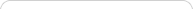##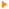Teaching with Sketchpad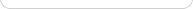•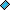- plays a video
•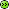- opens a web pageElementary school students bring two fundamental and powerful modes of thinking to school mathematics: the visual/qualitative and the numerical/analytic. Sketchpad’s interface combines these modes by providing visual representations of numbers and operations, and numerical interpretations of geometric shapes. This makes it possible to engage students with a variety of preferred learning styles, in mathematical activities across the curriculum and across grade levels.

## Geometry

Starting with geometry, students can use the basic tools in the Toolbox to create and manipulate points, circles, and lines. They can then create simple drawings—which they might color and animate—to form the basis for explorations and descriptions of geometric shapes. To develop both visual reasoning and geometric vocabulary, you might ask students to replicate given, or “found,” geometric designs. Young students often appreciate the accuracy of shapes drawn with the toolbox—as opposed to paper and pencil—and this appreciation prepares them for the mathematical idea of geometric construction.

Some of the most important ideas in geometry can be made accessible to students using only Sketchpad’s basic drawing tools. When do two lines intersect? How many circles pass through two points? How big can a triangle be? Give students a brief introduction to the Segment and Compass tools, and then set them free to pursue questions such as these. Students will quickly discover how to animate their shapes using the Display menu, providing them with images that are not only compelling, but that can draw their attention to important properties and relationships.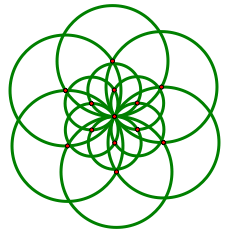## Transformations

After drawing shapes and designs, it’s natural to use transformations to extend student designs and create new ones. Students already know how to flip (reflect), slide (translate), and spin (rotate) physical objects. The Transform menu introduces them to mathematical definitions of these operations, and helps them extend their intuitive understandings to more formal ones. For example, let students explore the idea of an angle of rotation, starting with a simple shape of their own design. With that shape selected, students can choose Transform | Rotate and experiment with entering different angles of rotation into the Rotation dialog box. Point out how their experiments can create flowers, windmills, or even kaleidoscopes.

As they explore, Sketchpad helps students develop a geometric vocabulary for describing rotation and its elements, such as center of rotation, angle of rotation, image, and pre-image. Students can then use this vocabulary as they look for geometric relationships: What happens to the flower when the center of rotation changes?

## Measurement

Explorations with drawings or transformations naturally lead to using the Measure menu, which provides an additional means for students to develop their ability to describe and identify shapes.

Start by measuring the lengths of several segments, and asking students to use these measurements to describe which are longer, shorter, or of equal length. They can then use measurement as a means of classifying shapes. For example, given a sketch with different shapes on it—say, different types of triangles—students can use length or angle measurements to categorize the shapes into ones where all corresponding measurements are equal, ones where only some are equal, and ones where none are equal. These comparisons can be between shapes (where equal measurements can lead to ideas of congruence or similarity), or within a single shape (where you might investigate triangles with three equal side lengths, with exactly two equal side lengths, and with no equal side lengths).

Once students begin describing shapes purposefully, they can focus on some of the special properties of common shapes—such as parallel or perpendicular sides. They can use measurement to find or confirm these properties in pre-made shapes, or use the Construct menu to define new shapes based on these relationships themselves, beginning the process of making constructions that are geometrically robust.

## Prepared Models for Number Sense

Many prepared sketches are available that allow students to engage directly with mathematical models and manipulatives without having to use Sketchpad’s tools or commands—see the Sample Activities for some examples. While there are powerful learning experiences to be had constructing your own mathematics, prepared sketches can make an easy starting point for focusing on a specific topic or idea.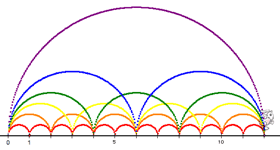For example, the Jump Along model allows students to explore various arithmetic operations in terms of “skip-counting,” or “jumping along” the number line. The model allows students to attach a visual meaning to operations such as 3 x 4, and motivate students to explore variations and extensions, such as by finding other pairs of number that arrive at the same spot on the number line—in this case, 12.

Prepared sketches often make use of parameters—named numeric values whose actual values students can change. When visual mathematical models incorporate changeable parameters, students can interact and experiment directly with variability, dependency, and consequence. For example, in a visual model of 50 apples divided into baskets, a student might change the “number of baskets” parameter from 10 to 5 to see what happens to the number of apples in each basket. Working with parameters in the early grades prepares students to understand the idea of a variable in later grades.

And of course, young students like puzzles and games. Many prepared sketches for elementary activities come with “make your own” challenges where students create puzzles for each other. This gives them greater ownership of their mathematics. While Sketchpad commands certainly contain some mathematical ideas outside the elementary school curriculum, young students often appreciate that the tools they use in Sketchpad are the very same ones that others use too—older students, teachers, even professional mathematicians. The skills and ways of thinking that students develop using Sketchpad in the early years will be useful as they continue in mathematics study in middle school, high school, and beyond.